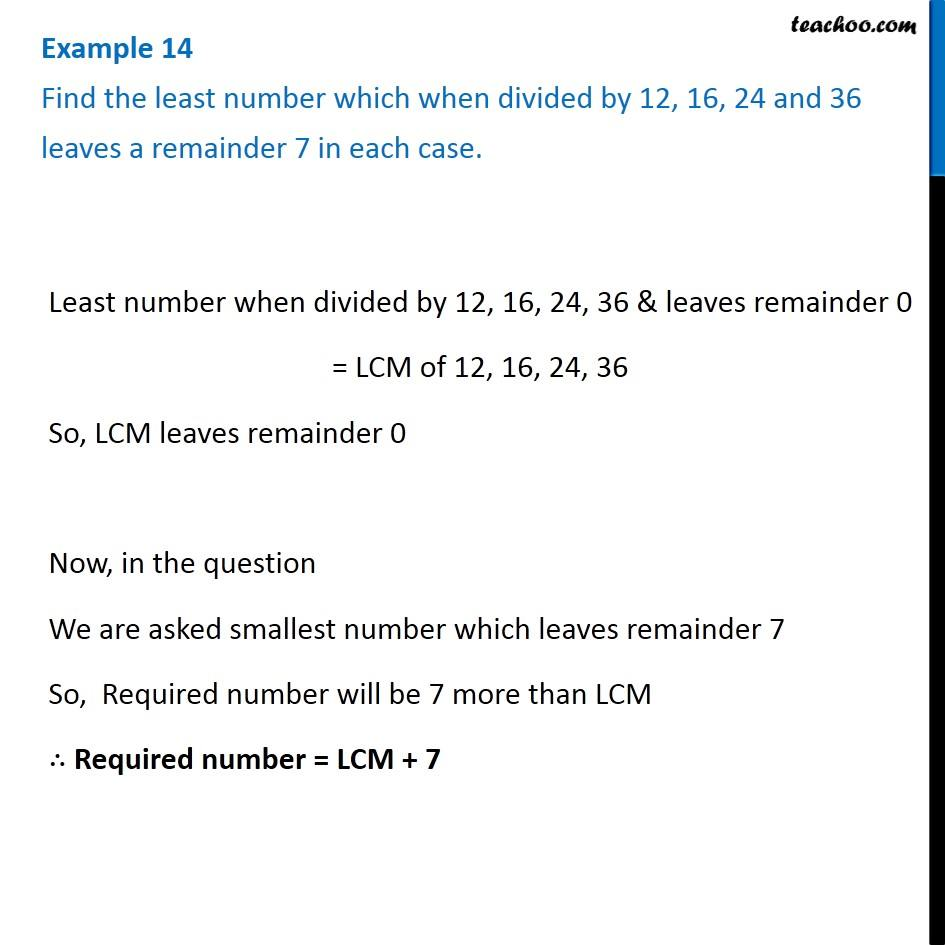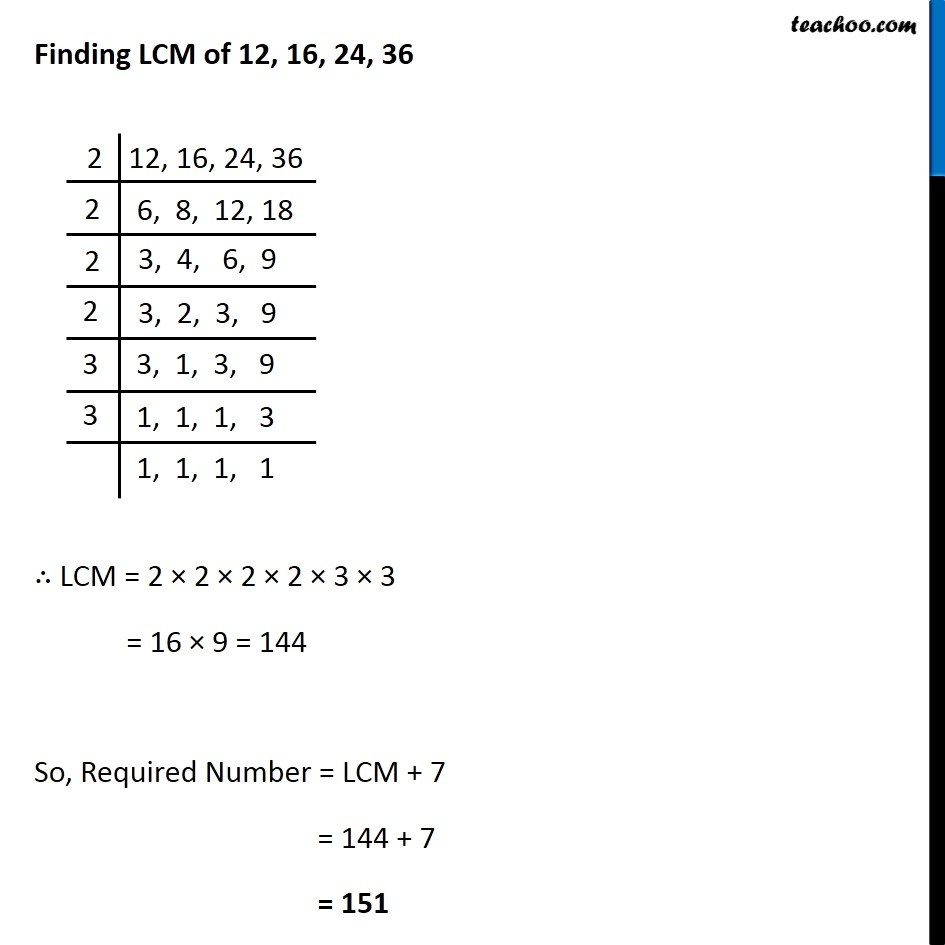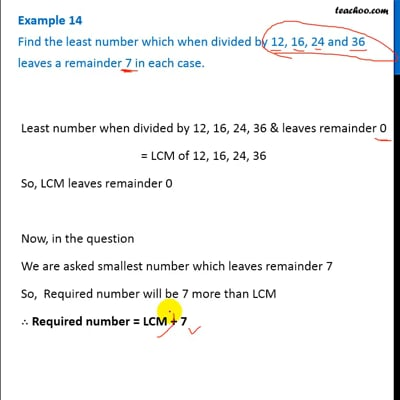Examples

Chapter 3 Class 6 Playing with Numbers
Serial order wiseThis video is only available for Teachoo black users

### Transcript

Example 14 Find the least number which when divided by 12, 16, 24 and 36 leaves a remainder 7 in each case. Least number when divided by 12, 16, 24, 36 & leaves remainder 0 = LCM of 12, 16, 24, 36 So, LCM leaves remainder 0 Now, in the question We are asked smallest number which leaves remainder 7 So, Required number will be 7 more than LCM ∴ Required number = LCM + 7 Finding LCM of 12, 16, 24, 36 ∴ LCM = 2 × 2 × 2 × 2 × 3 × 3 = 16 × 9 = 144 So, Required Number = LCM + 7 = 144 + 7 = 151